# ggdemetra: extending ggplot2 to perform seasonal adjustment with RJDemetra

#### 2022-11-11

`ggdemetra` is an extension of `ggplot2` to add seasonal adjustment statistics to your plots. The seasonal adjustment process is done with RJDemetra that is an R interface to JDemetra+, the seasonal adjustment software officially recommended to the members of the European Statistical System (ESS) and the European System of Central Banks. `RJDemetra` implements the two leading seasonal adjustment methods TRAMO/SEATS+ and X-12ARIMA/X-13ARIMA-SEATS.

There are 4 main functionalities in `ggdemetra` depending of what you want to add in the graphic:

• `geom_sa()`: to add a time series compute during the seasonal adjustment (the trend, the seasonal adjusted time series, etc.).
• `geom_outliers()`: to add the outliers used in the pre-adjustment process of the seasonal adjustment.
• `geom_arima()`: to add the ARIMA model used in the pre-adjustment process of the seasonal adjustment.
• `geom_diagnostics()`: to add a table containing some diagnostics on the seasonal adjustment process.

Note `ts` objects cannot be directly used in `ggplot2`. To convert `ts` or `mts` object to `data.frame`, you can use the `ts2df()` function. For example, the data `ipi_c_eu_df` used in this package is obtained by applying the `ts2df()`function to the `ipi_c_eu` data available in RJDemetra:

``ipi_c_eu_df <- ts2df(ipi_c_eu)``

All the functions have some common parameters and especially those to defined the seasonal adjustment method:

• `method` is the method used for the seasonal adjustment: X-13ARIMA (`method = "x13"`, the default) or TRAMO-SEATS (`method = "tramoseats"`).
• `spec` is the seasonal adjustment specification. It can be the name of pre-defined specification (see `?RJDemetra::x13` or `?RJDemetra::tramoseats`) or a user-defined specification created by `RJDemetra` (by `RJDemetra::x13_spec` or `RJDemetra::tramoseats_spec`).
• `frequency` is the frequency of the input time series. By default, the frequency is computed and a message is printed with the one chosen (use `message = FALSE` to suppress this message).

In the following examples, the data used is the French industrial production index. By default, the seasonal adjustment will then be processed with X-13ARIMA with a pre-defined specification `"RSA5c` (automatic log detection, automatic ARIMA and outliers detection and trading day and easter adjustment). However, in the industrial production the working day effect has more economic sense than the trading day effect and a gradual effect for easter does not make economic sense for the aggregated series. The specification that should be used with X-13ARIMA is `spec = RJDemetra::x13_spec("RSA3", tradingdays.option = "WorkingDays")`. If no new data or seasonal adjustment specification is specified (method or specification), these parameters is inherited from the previous defined: therefore you only need to specify this parameter once.

``````library(ggplot2)
library(ggdemetra)
p_ipi_fr <- ggplot(data = ipi_c_eu_df, mapping = aes(x = date, y = FR)) +
geom_line() +
labs(title = "Seasonal adjustment of the French industrial production index",
x = NULL, y = NULL)
p_ipi_fr``````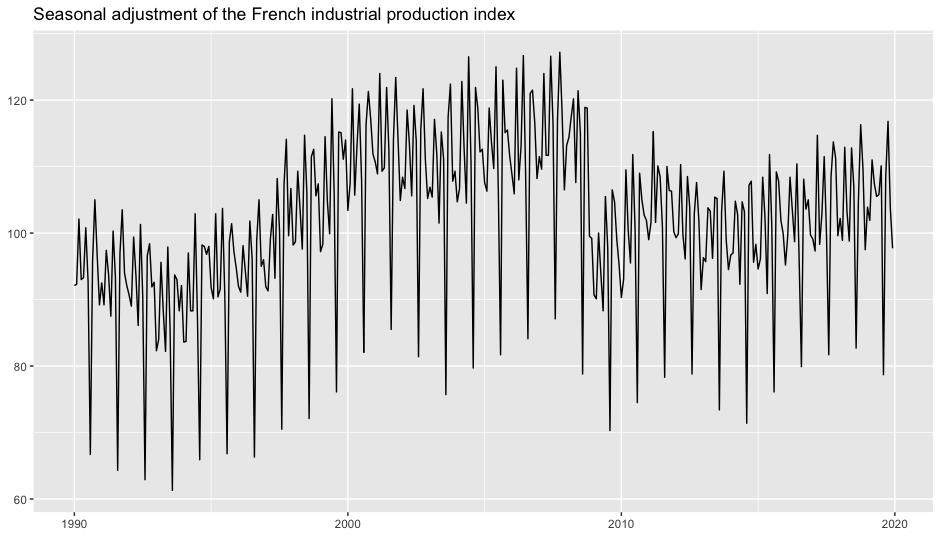By default `geom_sa()` adds the seasonal adjusted time series:

``````spec <- RJDemetra::x13_spec("RSA3", tradingdays.option = "WorkingDays")
p_ipi_fr +
geom_sa(color = "red",
spec = spec)
#> Frenquency used: 12``````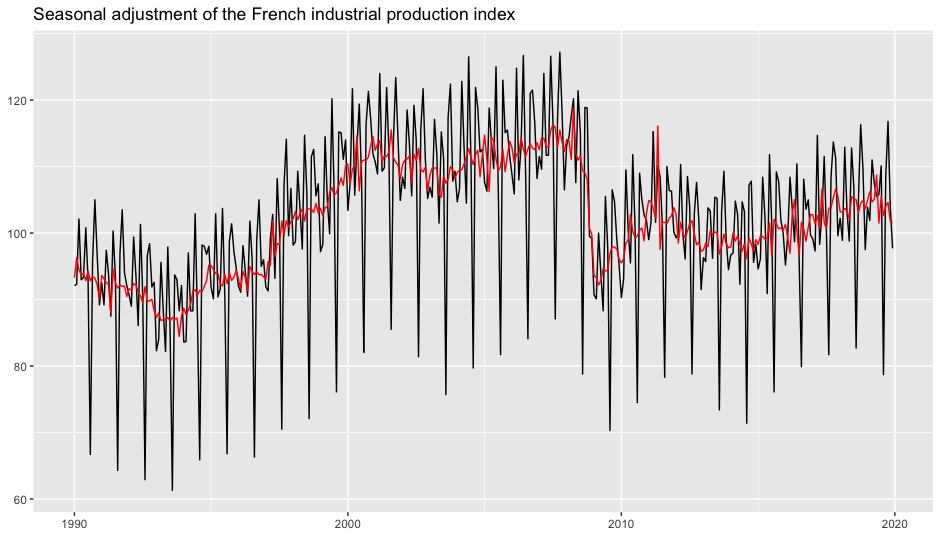To add other components of the seasonal adjustment, use the `component` parameter of `geom_sa()` (see `?RJDemetra::user_defined_variables()` for the availables parameters). For example, to add the forecasts of the input data and of the seasonal adjusted series:

``````p_sa <- p_ipi_fr +
geom_sa(component = "y_f", linetype = 2, message = FALSE,
spec = spec) +
geom_sa(component = "sa", color = "red") +
geom_sa(component = "sa_f", color = "red", linetype = 2)
p_sa``````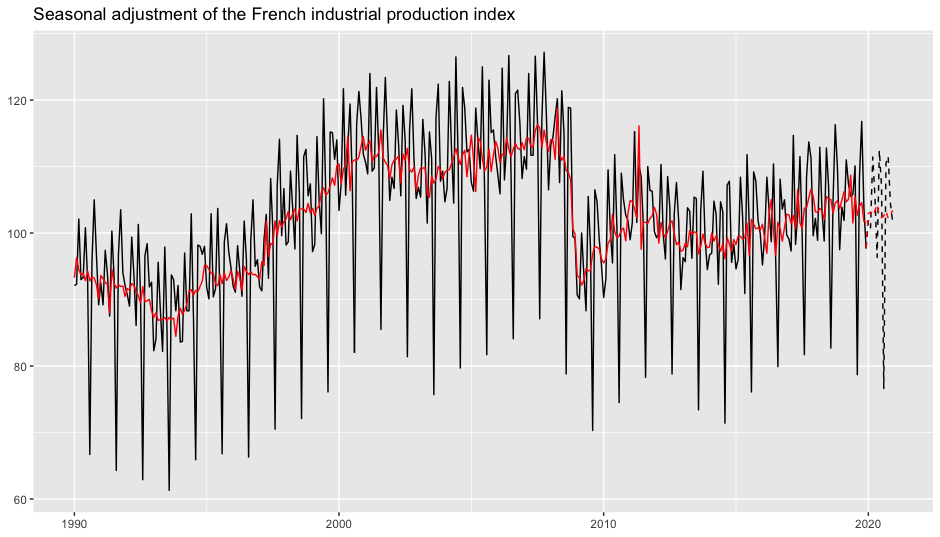# Add the outliers to the plot

There are four differents geometrics to add to the plot the names of the outliers used in the pre-adjustment process:

• `geom = "text"` (the default) adds directly the names of the outliers and `geom = "label"` draws a rectangle behind the names, making them easier to read.
• `geom = "text_repel"` and `geom = "label_repel"` do the same but text labels repel away from each other and away from the data points (see `?ggrepel::geom_label_repel`).

In our example, there are 3 outliers:

``p_sa + geom_outlier(geom = "label")``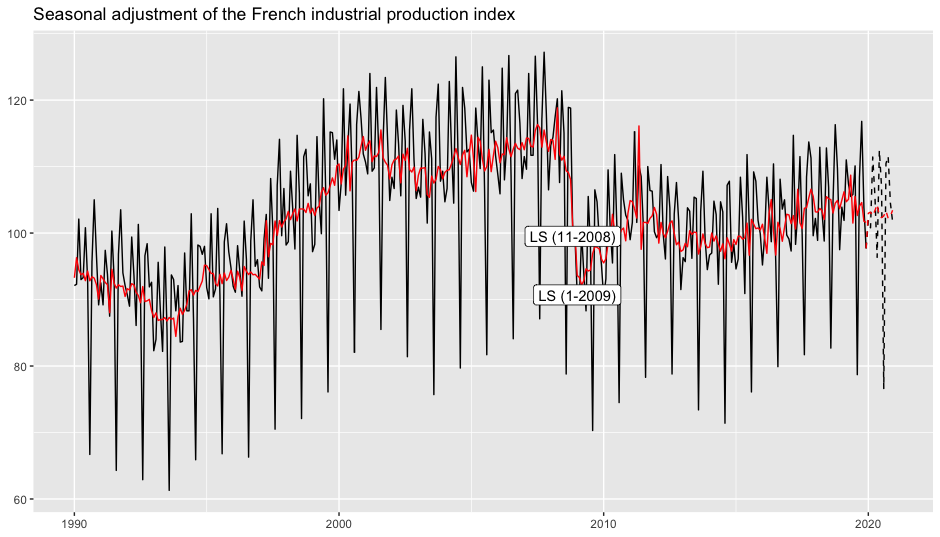They can be plotted in more readable way using the parameters of `ggrepel::geom_label_repel`:

``````p_sa +
geom_outlier(geom = "label_repel",
vjust = 4,
ylim = c(NA, 65), force = 10,
arrow = arrow(length = unit(0.03, "npc"),
type = "closed", ends = "last"))``````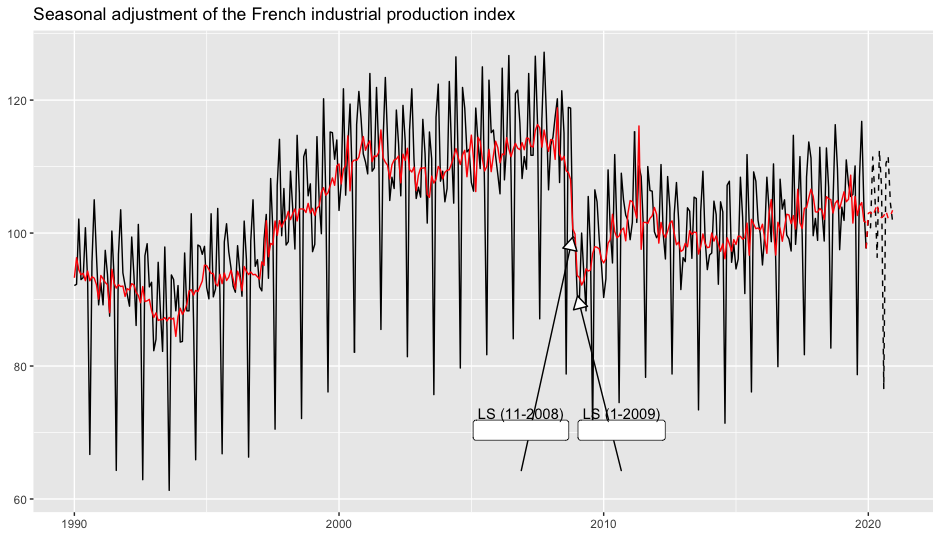Use the parameters `first_date` and `last_date` to only have the outliers in a precise time interval. For example, to only plot the outliers from 2009 use `first_date = 2009`:

``````p_sa +
geom_outlier(geom = "label_repel",
first_date = 2009,
vjust = 4,
ylim = c(NA, 65), force = 10,
arrow = arrow(length = unit(0.03, "npc"),
type = "closed", ends = "last"))``````